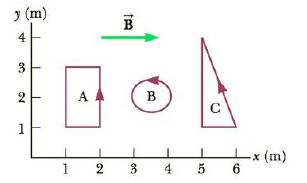Chapter 19, Problem 19CQ

Chapter
Section
Textbook Problem

A magnetic field exerts a torque on each of the current-carrying single loops of wire shown in Figure CQ19.19. The loops lie in the xy-plane, each carrying the same magnitude current, and the uniform magnetic field points in the positive x-direction. Rank the coils by the magnitude of the torque exerted on them by the field, from largest to smallest. (a) A, B, C (b) A, C, B (c) B, A, C (d) B, C, A (e) C, A, BFigure CQ19.19

To determine
The rank of the coils by the magnitude of the torque exerted on them by the field from largest to smallest.

Explanation

The magnitude of the torque exerted on a loop is given by,

τ=ΝΙΑΒsinθ

• Ν is the number of turns in the coil
• Ι is the current in the coil
• Α is the area of the coil
• Β is the magnetic field
• θ is the angle between the loop and the magnetic field

The loops are shown in the following figure.

All the loops have single turn, same current, same magnetic field and all are on the same plane. Hence the torque of the different loops will differ only due to the difference in their area.

The area of the loop A is given by,

SA=(1m)(2m)=2m2

The area of the loop B is given by,

SB=2π(0

Still sussing out bartleby?

Check out a sample textbook solution.

See a sample solution

The Solution to Your Study Problems

Bartleby provides explanations to thousands of textbook problems written by our experts, many with advanced degrees!

Get Started

Find more solutions based on key concepts